# Factoring PolynomialsPage 6

#### WATCH ALL SLIDES

Slide 36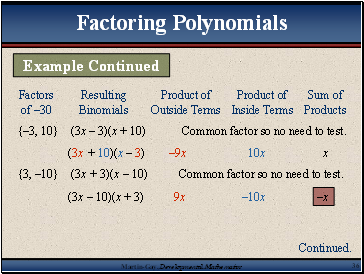{–3, 10} (3x – 3)(x + 10) Common factor so no need to test.

(3x + 10)(x – 3) –9x 10x x

{3, –10} (3x + 3)(x – 10) Common factor so no need to test.

Factoring Polynomials

Example Continued

Continued.

Slide 37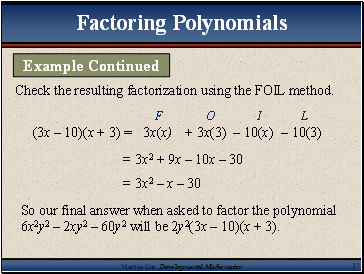Check the resulting factorization using the FOIL method.

(3x – 10)(x + 3) =

= 3x2 + 9x – 10x – 30

= 3x2 – x – 30

So our final answer when asked to factor the polynomial 6x2y2 – 2xy2 – 60y2 will be 2y2(3x – 10)(x + 3).

Factoring Polynomials

Example Continued

Slide 38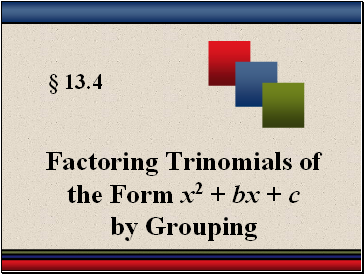§ 13.4

Factoring Trinomials of the Form x2 + bx + c by Grouping

Slide 39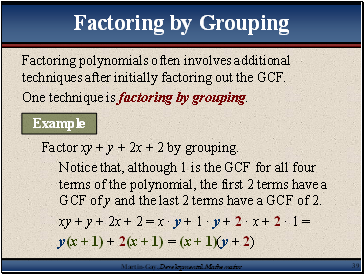## Factoring by Grouping

Factoring polynomials often involves additional techniques after initially factoring out the GCF.

One technique is factoring by grouping.

Factor xy + y + 2x + 2 by grouping.

Notice that, although 1 is the GCF for all four terms of the polynomial, the first 2 terms have a GCF of y and the last 2 terms have a GCF of 2.

xy + y + 2x + 2 = x · y + 1 · y + 2 · x + 2 · 1 =

y(x + 1) + 2(x + 1) = (x + 1)(y + 2)

Example

Slide 40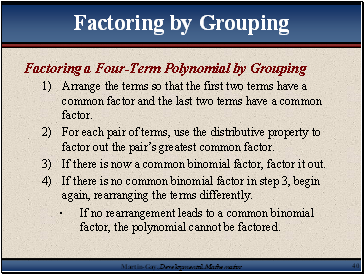Factoring a Four-Term Polynomial by Grouping

Arrange the terms so that the first two terms have a common factor and the last two terms have a common factor.

For each pair of terms, use the distributive property to factor out the pair’s greatest common factor.

If there is now a common binomial factor, factor it out.

If there is no common binomial factor in step 3, begin again, rearranging the terms differently.

If no rearrangement leads to a common binomial factor, the polynomial cannot be factored.

Factoring by Grouping

Slide 41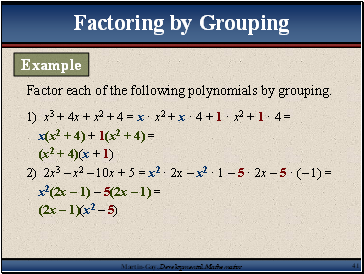1) x3 + 4x + x2 + 4 = x · x2 + x · 4 + 1 · x2 + 1 · 4 =

x(x2 + 4) + 1(x2 + 4) =

(x2 + 4)(x + 1)

2) 2x3 – x2 – 10x + 5 = x2 · 2x – x2 · 1 – 5 · 2x – 5 · (– 1) =

x2(2x – 1) – 5(2x – 1) =

(2x – 1)(x2 – 5)

Factor each of the following polynomials by grouping.

Factoring by Grouping

Example

Slide 42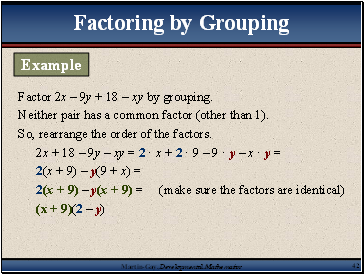Factor 2x – 9y + 18 – xy by grouping.

Neither pair has a common factor (other than 1).

So, rearrange the order of the factors.

2x + 18 – 9y – xy = 2 · x + 2 · 9 – 9 · y – x · y =

2(x + 9) – y(9 + x) =

2(x + 9) – y(x + 9) = (make sure the factors are identical)

(x + 9)(2 – y)

Factoring by Grouping

Example

Slide 43

Go to page:
1  2  3  4  5  6  7  8  9  10